# How far? How big ? How many ?

## Light - our measuring stick in space

How far does light travel in one second ? 300 000 kilometers, which equals seven times around the Earth.

Let's call this distance one light second. We can use this as a ruler to measure distances in space. Beyond the Sun and its planets we need a longer ruler, which is the distance light travels in one year. This is called a light year - it is 32 million times longer than a light second. Multiplying a light second by the number of seconds in a year, we can answer the question:
How far is a light year? 10 trillion kilometers (or more exactly, 9.5 x 1012 km).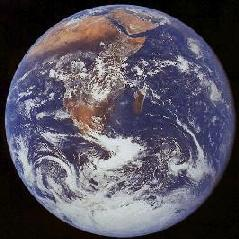The Earth, which is about 12000 kilometers in diameter (NASA).

## The Sun and its planets

• How far is the Moon from the Earth ? 1.3 light seconds.
• How far is the Earth from the Sun ? 500 light seconds or 8 light minutes.
• Which is the biggest of the Sun's planets ? Jupiter - it is 10 times the Earth's diameter.
• What is the mass of the Earth ? 6 000 000 000 000 000 000 000 000 kilograms.
• What is Jupiter's mass ? 300 times the mass of the Earth.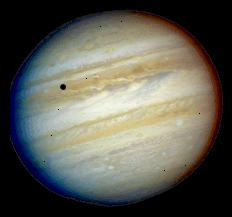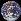The planet Jupiter with one of its moons, Io, casting a black shadow (NASA). The Earth, to the same scale as Jupiter (NASA)

• What is the Sun's diameter ? 110 times the Earth's diameter.
• What is the Sun's mass ? 330 000 times the mass of the Earth.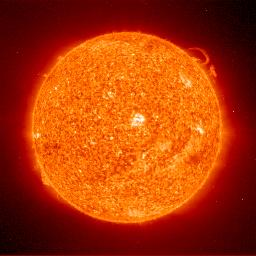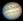The Sun seen in ultraviolet light by the SOHO spacecraft. (NASA/ESA). Jupiter to scale Earth to scale

• How big is the solar system (Sun and planets) ? 10 light hours from one side to the other.
• Are there planets around other stars ? At least 80 had been found by January 2002. They were found by detecting very slight wobbles in the motion of the parent stars. One was confirmed by measuring the dimming the light of the parent star as the planet passed in front of the star.

## The Stars

• How far is the nearest star (after the Sun) ? Proxima Centauri is 4.3 light years away. It is a dim red star orbiting the bright double star, Alpha Centauri. These nearest stars are 270 000 times further from the solar system than the Sun is from the Earth.
• What are the smallest stars ? Neutron stars collapse to about 15 kilometers in diameter. We observe them as pulsars.
• What are the biggest stars ? Red Giant stars swell up to 500 times the diameter of the Sun. Betelguese, Antares and Aldebaran are well known red giant stars.

## Our Galaxy, the Milky Way

• How far is the Sun from the middle of our galaxy, the Milky Way ? 30 000 light years.
• How big is the Milky Way ? 100 000 light years across. This is 100 million times the diameter of Pluto's orbit.
• How many stars are there in the Milky Way ? About 100 billion (= 1011).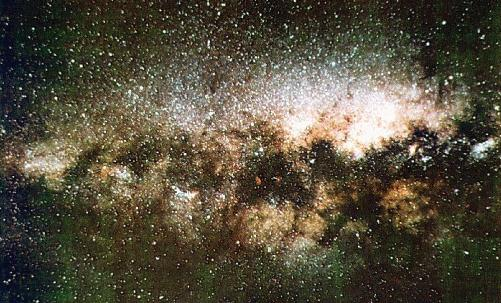Stars and dust clouds towards the center of the Milky Way. Stars form half the detectable mass of the Milky Way. The other half is in the form of gas and dust.

## The Universe

• How far away is the nearest galaxy ? The newly discovered dwarf galaxy in Sagittarius, only 60 000 light years away, is spiralling into the Milky Way. The nearest large spiral galaxy like the Milky Way is the Andromeda galaxy, about 2.7 million light years away.
• How many galaxies are there in the visible universe ? Over 2 million have been counted, but there could be about 100 000 million.
• How big is the visible universe ? About 15 000 million light years.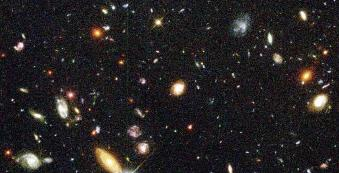Distant galaxies seen by the Hubble Space Telescope (STSCI/NASA).

More about Space and Time, Stars and Planets